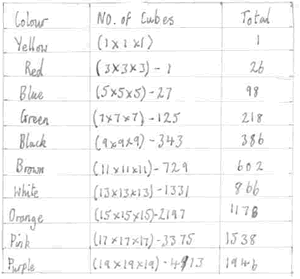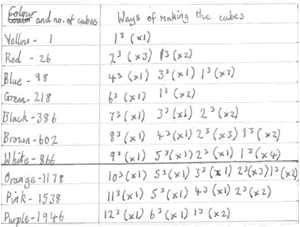#### You may also like### Cubes

How many faces can you see when you arrange these three cubes in different ways?### Pebbles

Place four pebbles on the sand in the form of a square. Keep adding as few pebbles as necessary to double the area. How many extra pebbles are added each time?### Bracelets

Investigate the different shaped bracelets you could make from 18 different spherical beads. How do they compare if you use 24 beads?

# Cubes Within Cubes

##### Age 7 to 14 Challenge Level:

This problem was very well answered by Martha from Tattingstone School:

We worked out this problem by finding out how many cubes would cover 1 (yellow) cube, which was $3\times 3\times3$ (27). Then we found that a $5\times 5\times5$ cube would be the next one and so on. The trouble was that the $3\times 3\times3$ cube was a totally different cube than the $1\times 1\times1$ cube. So with the 27 we had to take away 1 as the $3\times 3\times3$ was only the skin. So in order to get the skin each time it would be $5\times 5\times5$ - $3\times 3\times3$ etc. Here are my results:To answer the second part of question, when this large cube is broken up, Martha drew another table which clearly shows how each colour was made into the largest possible cubes:Martha continues:

The only problem with our results is that from the orange cubes downwards (in the table) they are all over 1000 and it said in the question "up to 1000 of each colour".

So, in answer to the three questions that were posed, Martha says:

The colour of the largest cube that was made was white.
The colour with no $1\times 1\times1$ cubes in it was black.
The colour with the most cubes made out of it including the $1\times 1\times1$ cubes was blue.

Bronya, also from Tattingstone sent in a good solution too - well done.